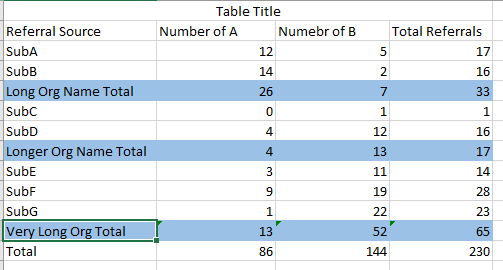I have some data that looks like this:

data tabletest;
informat referral_total \$50. referral_source \$20.;
infile datalines delimiter='|';
input referral_total referral_source TypeA TypeB ;
datalines;
Long Org Name | SubA | 12 | 5
Long Org Name | SubB | 14 | 3
Longer Org Name | SubC | 0 | 1
Longer Org Name | SubD | 4 | 12
Very Long Org | SubE | 3 | 11
Very Long Org | SubF | 9 | 19
Very Long Org | SubG | 1 | 22
;
run;

I have some preliminary code to add subtotals by the referral_total values.

column referral_total referral_source TypeA TypeB;
define referral_total / group ;
define referral_source / group;
define TypeA / sum ' ';
define TypeB / sum ' ';
break after referral_total / summarize style={background=lightblue font_weight=bold };
rbreak after /summarize;
compute referral_total;
if _break_ = 'referral_total' then
do;
referral_total = catx(' ', referral_total, 'Total');
end;
else if _break_ in ('_RBREAK_') then
do;
referral_total='Total';
end;
endcomp;
run;

1. How do I add a column that is a sum of TypeA + TypeB?  This would be named Total Referrals.

2. How do I change and format the labels of the header in the table? (see example desired output)

-Add a spanned row with the Table Title

-Have Referral Source, Number of A, Number of B as columns with the correct labels and not the name of the variable from the dataset.3 REPLIES 3

PROC TABULATE does a lot this by default. Are you set on using PROC REPORT?

@appleorange wrote:

I have some data that looks like this:

data tabletest;
informat referral_total \$50. referral_source \$20.;
infile datalines delimiter='|';
input referral_total referral_source TypeA TypeB ;
datalines;
Long Org Name | SubA | 12 | 5
Long Org Name | SubB | 14 | 3
Longer Org Name | SubC | 0 | 1
Longer Org Name | SubD | 4 | 12
Very Long Org | SubE | 3 | 11
Very Long Org | SubF | 9 | 19
Very Long Org | SubG | 1 | 22
;
run;

I have some preliminary code to add subtotals by the referral_total values.

column referral_total referral_source TypeA TypeB;
define referral_total / group ;
define referral_source / group;
define TypeA / sum ' ';
define TypeB / sum ' ';
break after referral_total / summarize style={background=lightblue font_weight=bold };
rbreak after /summarize;
compute referral_total;
if _break_ = 'referral_total' then
do;
referral_total = catx(' ', referral_total, 'Total');
end;
else if _break_ in ('_RBREAK_') then
do;
referral_total='Total';
end;
endcomp;
run;

1. How do I add a column that is a sum of TypeA + TypeB?  This would be named Total Referrals.

2. How do I change and format the labels of the header in the table? (see example desired output)

-Add a spanned row with the Table Title

-Have Referral Source, Number of A, Number of B as columns with the correct labels and not the name of the variable from the dataset.I think so?  I'm relatively new to proc report/tabulate, but this table will be one of many tables in a report with ODS formatting to PDF.

Well, changing your data structure slightly makes this a lot simpler. That's the first step I do, make it a long data set so TYPE is a variable that holds values of A/B and add a variable called VALUE that holds the amounts.

Here's the PROC TABULATE solution. Notice the use of the ALL keywords that control which totals you get and where they go. If you put ALL before the variable name for example, totals will be your first row.

``````data long;
set tabletest;
Type='A';
Value = typeA;
output;
Type='B';
value = typeB;
output;
drop typeA typeB;
run;

proc tabulate data=long;
class referral_total referral_source type;

var value;
table (referral_total*(referral_source all) all), (type all)*value=''*sum=''*f=8.;
run;``````

Here's a decent paper on the topic if you need to understand the details or what anything in particular is doing.

http://support.sas.com/resources/papers/proceedings11/260-2011.pdf

Discussion stats
• 3 replies
• 762 views
• 0 likes
• 2 in conversation